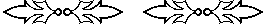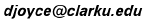## Qin Jiushao’s algorithm for finding one

Qin Jiushao (1202-1261) was a Chinese mathematician who wrote Shushu jiuzhang (Mathematical Treatise in Nine Sections). In it he has a general method for solving simultaneous linear congruences (the Chinese Remainder Theorem).

#### Summary of the general method

Before looking at Qin Jiushao’s method of finding one, let’s quickly review the general method of solving simultaneous linear congruences. Let’s take the following system of three linear congruences as our example.

 N ≡ 7 (mod 9) N ≡ 13 (mod 23) N ≡ 1 (mod 2)

Thus, we’re looking for one number N such that when you divide N by 9 you get a remainder of 7, when you divide N by 23 you get a remainder of 13, and when you divide N by 2 you get a remainder of 1 (i.e., N is odd).

Step 1.
Compute the product of all the moduli. 9 times 23 times 2, which is 414.
Step 2.
For each modulus, compute the product of the remaining moduli. For the first, we have 23 times 2 is 46. For the second, we have 9 times 2is 18. For the third, we have 9 times 23 is 207. (These could also be found by dividing the product computed in step 1 by the modulus under consideration.)
Step 3.
For each of the numbers we just found, reduce it modulo the given modulus. For the first, 46 modulo 9 gives 1. For the second, 18 modulo 23 is 18. For the third, 207 modulo 1 is 1.
Step 4.
This is the step called finding one. For each of those numbers found in step 3, find the reciprocal modulo the given modulus. For the first, we need to solve the congruence x so that 1x ≡ 1 (mod 9), but that x is 1. For the second, we need to solve the congruence 18x ≡ 1 (mod 23). Searching quickly gives x=9, so 9 is the reciprocal of 18 modulo 23. For the third, the reciprocal modulo 2 of 1 is 1.
Step 5.
To get N sum three numbers. The first is 7 times 46 times 1, which equals 322, where the 7 is the constant in the first congruence, 46 is the number computed in step 2, and 1 is the number computed in step 4. The second is 13 times 18 times 9, which equals 2106. The third is 1 times 207 times 1 which is 207. Adding those three numbers together gives N = 322+2106+207 = 2635.
Step 6.
The answer in step 5 can be reduced modulo the product computed in step 1 to get the final answer. Modulo 414, 2635 reduces to 151. Thus, N = 151 is the smallest positive solution to the system of three simultaneous linear congruences.

#### Qin Jiushao’s method of finding one

In step 4 in the preceding algorithm, we need to find a solution to the congruence

ax ≡ 1 (mod b)

where a and b are relatively prime integers.

For small a and b this can be done by a quick search. For instance, to solve 5x ≡ 1 (mod 21), just look among the multiples of 5 for one that is 1 more than a multiple of 21. Multiples of 5 are 5, 10, 15, 20, 25, 30, 35, 40, 45, 50, 55, 60, 65, 70, 75, 80, 85, etc., while multiples of 21 are 21, 42, 63, 84, etc. Since 85 is 1 more than 84, a solution is x = 17 (since 5 times 17 is 85). Note that 17 is the smallest positive solution, but you can find larger ones by repeatedly adding 21 to 17: 38, 59, 80, etc. Generally, the smallest positive solution is desired.

For large a and b a search like that would be very laborious. Imagine trying to solve 168x ≡ 1 (mod 295). Here’s an algorithm to find the solution quickly. First the algorithm will be outlined, then we’ll try to figure out why it works.

Start by placing the numbers 1, 0, 168, and 295 in a square like this:

 1 168 0 295

Note: the Chinese worked on a counting board with rods to indicated numbers, so this algorithm was all done in the same square. Here, you’ll see the different configurations of numbers in that square as the computations proceed.

Look in the right column. Subtract the smaller number 168 from the larger 295, and in the left column add the number to the left of the smaller number, namely 1, to the number to the left of the larger number, namely 0. You get

 1 168 1 127

Next subtract 127 from 168 while adding the 1 in the lower left to the 1 in the upper left:

 2 41 1 127

Now repeatedly subtract 41 from 127, and at the same time add the lower left 1 to the upper left entry, until the upper right entry is less than 41.

 2 41 3 86
 2 41 5 45
 2 41 7 4

Actually, you can do this faster with division, which after all is repeated subtraction. Divide 41 into 127. You get a quotient of 3 and a remainder of 4. Replace the 127 by the remainder 4, and multiply the quotient 3 by the upper left entry 2 to get 6, and add that 6 to the lower left entry 1 to get 7.

Next, to repeatedly subtract 4 from 41. Here, it’s much better to divide instead: 41 divided by 4 gives a quotient of 10 and a remainder of 1. Replace the 41 by 1, and add the product of 7 and 10 to the 2 in the upper left corner to put 72 there.

 72 1 7 4

Stop here because you have a 1 in the upper right corner. To its left is 72, the answer we’re looking for. Let’s check to see that our answer x = 72 is actually a solution to the congruence 168x ≡ 1 (mod 295). Now, 168 times 72 is 12096. And 12096 divided by 295 is 41 with a remainder of 1. It worked!

(What do you do if you get a 1 in the lower right corner? Subtract it from the upper right corner until you get a 1 there. This you can always do. Alternatively, you can stop there and take the negation of the lower left corner as your answer.)Now to answer the question: why does the algorithm work? First, notice that what we did in the right column was to apply the Euclidean algorithm to find the greatest common divisor of 168 and 295. Since they were assumed to be relatively prime, that algorithm ended with their greatest common divisor of 1.

Solving the congruence 168x ≡ 1 (mod 295) is the same as solving the equation

168x = 1 + 295y.

We can follow the steps taken in the Euclidean algorithm in the right column by introducing new variables. Let x = y + z. Then 168y + 168z = 1 + 295y. Therefore,

168z = 1 + 127y.

Next, let y = z + a. Then 168z = 1 + 127z + 127a. Therefore,

41z = 1 + 127a.

Next, take z = 3a + b. Then 123a + 41b = 1 + 127a. Therefore,

41b = 1 + 4a.

Finally, let a = 10b + c. Then 41b = 1 + 40b + c. Therefore,

b = 1 + 4c.

Now, choosing c to have any value you like, and working your way back through the variable to x, you’ll get a solution. The easiest solution is to take c to be 0, and you’ll find x is 72, the solution found by Qin Jiushao’s algorithm.

The left column actually determines what x in terms of the later variables. Here’s how. Note how the coefficients are just the entries in the left column of the squares.

 x 1, 0 = z + y 1, 1 = z + (z + a) = 2z + a 2, 1 = 2(3a + b) + a = 2b + 7a 2, 7 = 2b + 7(10b + c) = 72b + 7c 72, 7

Finally, since b = 1 + 4c, we get x = 72(1 + 4c) + 7c, so

x = 72 + 295c

Everything done here for the specific congruence 168x ≡ 1 (mod 295) applies just as well to the general congruence ax ≡ 1 (mod b) where a and b are relatively prime integers. Relative primality is needed to assure that the algorithm eventually reaches a 1 in the right column where the greatest common divisor of a and b is being constructed.

Here are some progressively harder problems to work on. The smallest positive solutions are given so you can check your work.

 9 x ≡ 1 (mod 20) x = 9 20 x ≡ 1 (mod 9) x = 5 14 x ≡ 1 (mod 45) x = 29 88 x ≡ 1 (mod 105) x = 37 214 x ≡ 1 (mod 287) x = 114

You can make up your own problems ax ≡ 1 (mod b). Just make sure the a and b are relatively prime so that a solution exists. If they’re not, then you won’t be able to find 1.Nov 2000, Nov 2006
David E. Joyce